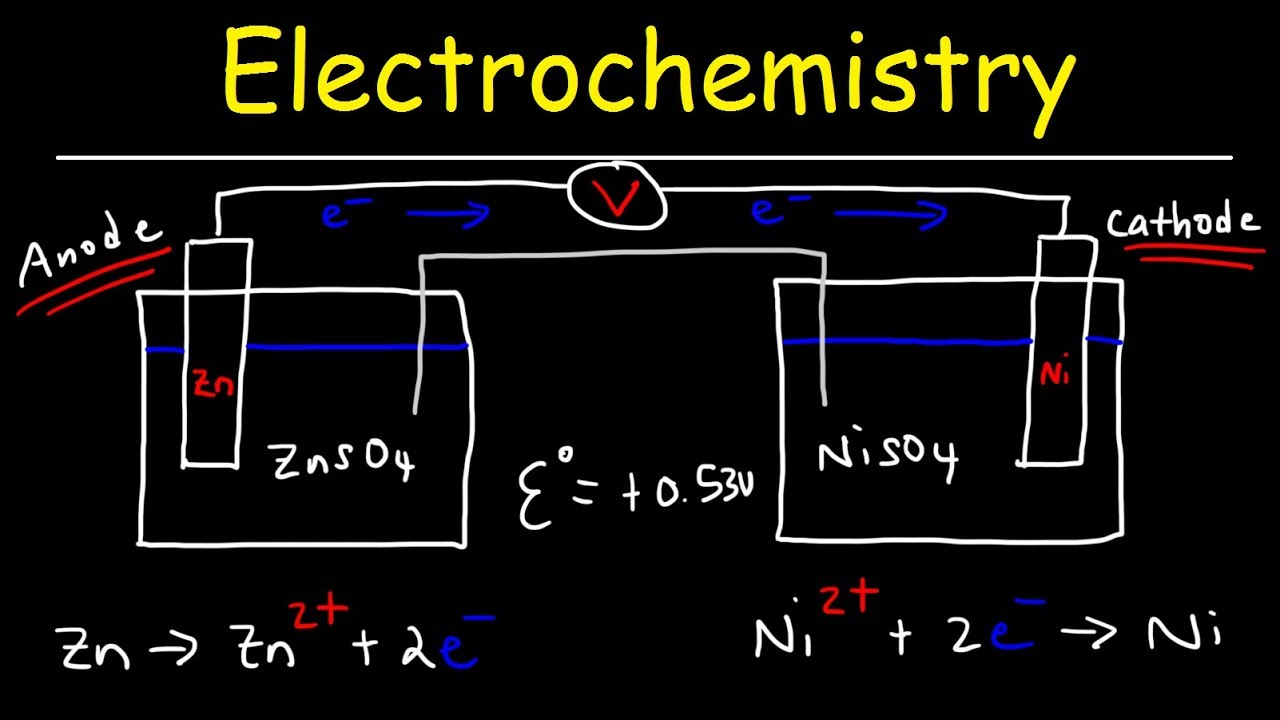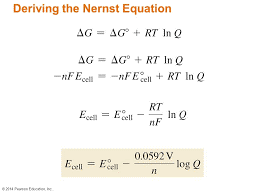# Electrochemistry Introduction, Principle, Conductivity, Factors affecting electrolytic conductance,Debye – Huckel and Onsager equation, Kohlraush’s law, Nernst Equation etc..?

0

### Electrochemistry:

The branch of chemistry that deals with the electrical energy transport and the inter conversion of electrical and chemical energy is called electrochemistry.

When an electric field is applied to such an electrolytic solution, the ions present in the solution carry charge from one electrode to another electrode and thereby they conduct electricity. The conductivity of the electrolytic solution is measured using a conductivity cell.

It obeys Ohm’s law i.e., at a constant temperature, the current flowing through the cell (I) is directly proportional to the voltage across the cell(V).

i.e., IαV

I = V / R

V = IR.### Resistivity:

The resistivity is defined as the resistance of an electrolyte confined between two electrodes having unit cross sectional area and are separated by a unit distance. The ratio (l/A) is called the cell constant.

Unit of resistivity is ohm metre.

R α l/A

R = ρ l/A

Where ρ is called the specific resistance which depends on the nature of the electrolyte.

### Conductivity:

The reciprocal of the resistance (1/R) gives the conductance of an electrolytic solution.

The SI unit of conductance is Siemen (S).

C = 1/R.

The reciprocal of the specific resistance (1/ρ) is called the specific conductance. It is represented by the symbol Kappa (к).

К = C. (l/A)

The specific conductance is defined as the conductance of a cube of an electrolytic solution of unit dimensions. The SI unit of specific conductance is Sm-1.

### Molar conductivity:

A conductivity cell in which the electrodes are separated by 1m and having V m3 of electrolytic solution which contains 1 mole of electrolyte. The conductance of such a system is called the molar conductance (λm).

READ  First Law of Thermodynamics Introduction, Principle, Types of Systems, Properties, Zeroth law of Thermodynamics ?

λm = К × V.

### Equivalent conductance (λ):

Equivalent conductance is defined as the conductance of ‘V’ m3 of electrolytic solution containing one gram equivalent of electrolyte in a conductivity cell in which the electrodes are one metre apart.

λ= К × 10-3/ N

where К the specific conductance and N is the concentration of the electrolytic solution expressed in normality.

### Factors affecting electrolytic conductance:

• Solvent of higher dielectric constant show high conductance in solution.
• Conductance is inversely proportional to the viscosity of the medium.
• If the temperature of the electrolytic solution increases, conductance also increases.
• Molar conductance of a solution increases with increase in dilution.

### Debye – Huckel and Onsager equation:

At infinite dilution, the interaction between the ions in the electrolyte solution is negligible. The influence of ion – ion interactions on strong electrolyte’s conductivity was studied by Debye and Huckle. For a uni-univalent electrolyte the Debye Huckel and Onsager equation is given below.

λm = λm – ( A+Bλm) √C

where A and B are the constants which depend only on the nature of the solvent and temperature.

### Kohlraush’s law:

According to Kohlraush’s law, the molar conductivity at infinite dilution for a electrolyte represented by the formula Ax By is given below.

m) AxBy = X (λm) Ay+ + y (λm) Bx-

### Applications of Kohlrausch’s law:

• Calculation of molar conductance at infinite dilution of a weak electrolyte.
• Calculation of degree of dissociation of weak electrolytes.
• Calculation of solubility of sparingly soluble salts.

### Electrochemical Cell:

Electrochemical cell is a device which converts chemical energy into electrical energy and vice versa. It consists of two separate electrodes which are in contact with an electrolyte solution. It is mainly classified into two types.

Galvanic cell (Voltaic cell)

It is a device which converts chemical energy into electrical energy. It is commonly known as a battery.

Electrolytic cell

It is a device which converts electrical energy into chemical energy.

emf of a cell

The force that pushes the electrons away from the anode and pulls them towards cathode is called the electromotive force or the cell potential.

READ  What is Sodium Carbonate, Balancing equation , uses and Videos etc...

### Nernst Equation:

Nernst equation is the one which relates the cell potential and the concentration of the species involved in an electrochemical reaction.

Let us consider an electrochemical cell for which the overall redox reaction is

xA + yB                   lC + mD

The reaction quotient Q for the above reaction is given below.

Q = [C]l [D]m / [A]x [B]y

We have learnt that,

∆G = ∆G + RT ln Q### Electrolytic cell and electrolysis:

Electrolysis is process in which the electrical energy is used to cause a non-spontaneous chemical reaction to occur; the energy is often used to decompose a compound into its elements. The device which is used to carry out the electrolysis is called the electrolytic cell.

First law

READ  Basics of green chemistry and day to day life..?

The mass of the substance liberated at an electrode during electrolysis is directly proportional to the quantity of charge passed through the cell.

i.e., m α Q

We know that the charge is related to the current by the equation I = Q /t

m α It

m = Z It

where Z is known as the electro chemical equivalent of the substance produced of the electrode.

Second law

When the same quantity of charge is passed through the solutions of different electrolytes, the amount of substances liberated at the respective electrodes are directly proportional to their electrochemical equivalents.

m α Z

### Fuel Cell:

The galvanic cell in which the energy of combustion of fuels is directly converted into electrical energy is called the fuel cell.